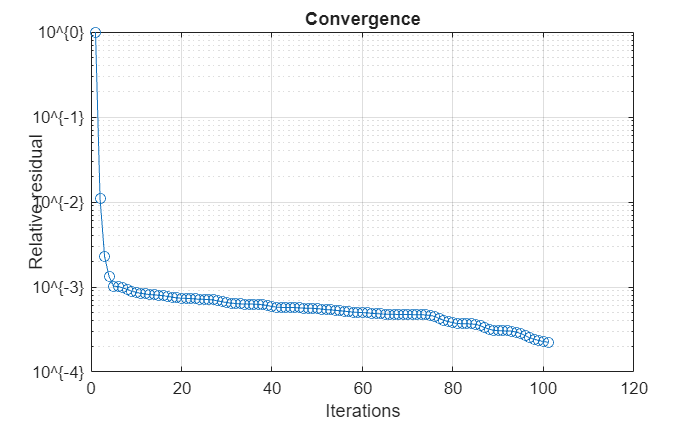# convergence

Calculate and plot convergence of FMM solver

## Syntax

``convergence(hsolver)``

## Description

example

````convergence(hsolver)` calculates and plots convergence of the fast multipole method (FMM) solver defined in `hsolver`. The function calculates the convergence over the iterations and relative residual specified in the `hsolver` object.```

## Examples

collapse all

Design a default parabolic reflector antenna.

`m = reflectorParabolic;`

Set the solver type of the parabolic reflector antenna to FMM.

`m.SolverType = 'FMM';`

Calculate the impedance of the parabolic reflector antenna at 10 GHz.

`Z = impedance(m,10e9);`

Access the FMM solver and set the relative residual to 1e-3.

```s = solver(m); s.RelativeResidual = 1e-3;```

Calculate and plot the convergence of the FMM solver for the parabolic reflector antenna.

`convergence(s)`## Input Arguments

collapse all

Fast multipole method (FMM) solver, specified as a `solver` object.

## Version History

Introduced in R2021b